## How Alteryx can solve functions with an Iterative Macro (plus a bit of a maths lesson...)

by Nicholas Bowskill

There are four types of macro in Alteryx; a standard macro, which just runs once inside a workflow; a batch macro, with runs multiple times in a workflow and outputs after each run; a location optimiser macro, which can be used to find optimal location (but apparently not all that used); and an iterative macro which runs inside the workflow over and over either a set amount of times or until a condition is met. Having just come from a maths degree I had come across the use of iterative functions being used to solve equations in graphical calculators, so decided to give it a go in Alteryx.

For those that don’t know, iteratively solving an equation involves rearranging the equation in question into a form where the variable is on both sides but on its own one of the sides. For example Newton’s method reduces the equation x^2=S to x=0.5*(x + S/x) (see https://en.wikipedia.org/wiki/Methods_of_computing_square_roots for more details) and the right hand side of the equation is used as the new value for x after a number has been plugged in. So to find the answer to x^2 = 4, starting at x=1 (which is fine to be any number we wish) the process goes: x=1, x=2.5, x=2.05, x=2.0006, etc. So we can see it gets very close to 2 very quickly.

For the square rooting example we just need to set up the macro input to expect a number that it is going to try to find the square root to and also a number to start iterating from, like so: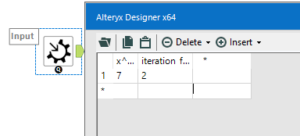After making sure that these inputs are in a number format able to go into decimals then the iterative function can be put into a Formula tool. We will use this formula tool to create two iterations after one another, the first to overwrite the ‘iteration first’ number and the second to use this overwritten number in the equation. As the second is run after the first it will be slightly closer to the real answer and can be compared against it; creating a new field called ‘difference’ with the equation ABS([iteration first] – [iteration second]) will find the absolute difference between the two numbers, it is this difference which will be the basis of our condition for the iteration to stop running. Here is what the Formula tool should look like: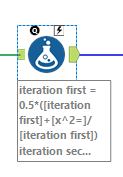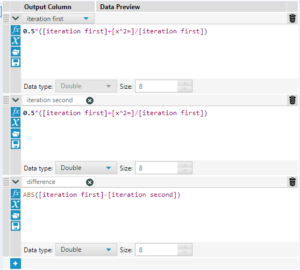As the iteration runs the two values will get closer and closer to each other, so the ‘difference’ can be used as a degree of accuracy for the macro. Adding in a Filter to the workflow with the condition of [difference]<0.000001 will mean that the macro will run until the answer in the output is less than 0.000001 distance from the true answer.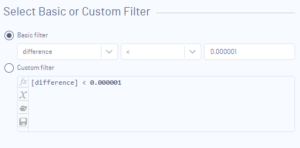Clicking on a blank area on the workflow and selecting Workflow in the Workflow – Configuration tab on the left hand side of the screen we can set the macro to be iterative.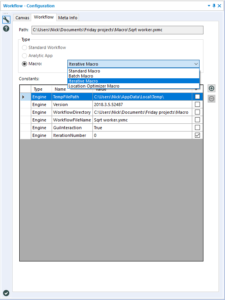If we add a macro output on to the false output of the filter then call the output ‘Go again’, or something else to know this is the output needed to repeat the process then the Interface Designer of the workflow (Ctrl+Alt+D as the keyboard shortcut) can be used to set the iteration input as the one set up earlier and the output as ‘Go again’. This means that the macro will start at whatever is fed into it and then will repeat for each time the condition on the difference is not met. Also setting the limit of iterations to something fairly high will allow the iteration to get to difficult answers and also not crash itself.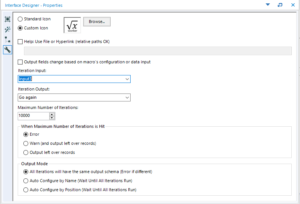Out of the true output of the filter only the answer given will ever come through so some clean up can be done. Changing the name of ‘iteration second’ to ‘Answer’ and using a Select to get rid of the fields that are no longer needed before giving this route a macro output will tidy up the results to be output into the overlying workflow. This should give you a simple looking workflow with a nice mathsy functionality to it: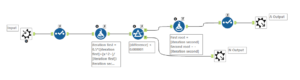This macro can then be hidden in a standard macro which sets up the data in the correct way for the flow to make the input look a little nicer, but this iterative macro is a nice logical way to see how iterative macros work which certainly taught me a lot about them whist making it and hopefully can help you too. Or at least impress your friends with a lovely square rooter (or not….)!Nicholas Bowskill

Fri 01 Feb 2019

Sun 20 Jan 2019

Sat 19 Jan 2019

Tue 08 Jan 2019